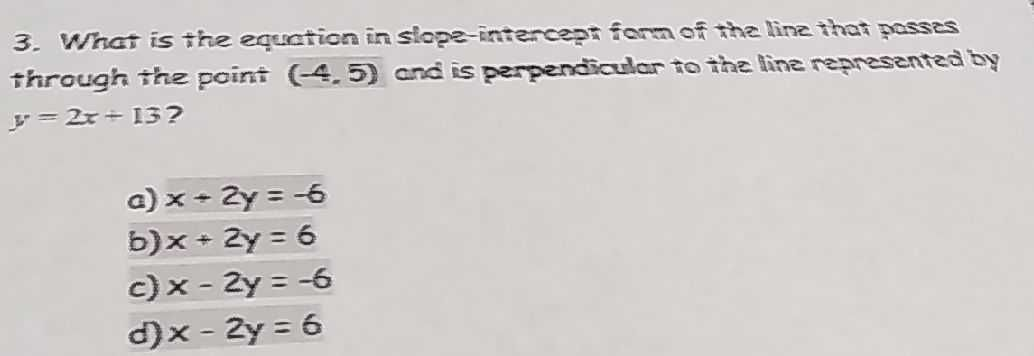### ¿Todavía tienes preguntas de matemáticas?

Pregunte a nuestros tutores expertos
Algebra
Pregunta3. What is the equation in siope-intencept form of the linz that passes through the point $$( - 4,5 )$$ and is perpendicular to the line representeat by

$$y = 2 x + 13 ?$$

a) $$x - 2 y = - 6$$

b) $$x + 2 y = 6$$

c) $$x - 2 y = - 6$$

d) $$x - 2 y = 6$$